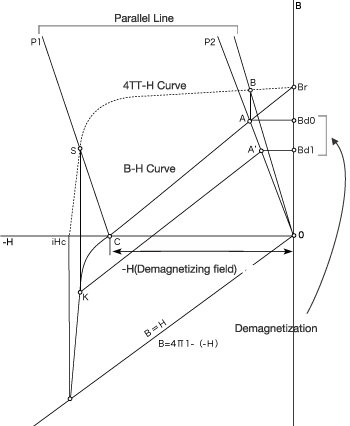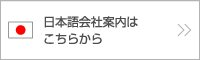Home > Basic Knowledge > Demagnetization by Effect of an External Magnetic Field## Demagnetization by Effect of an External Magnetic Field

The operating point moves by placing the magnetic substance close to the magnetized magnet or an external magnetic field is added to the magnetized magnet, and the magnetism changes. In retrorse, if the external magnetic field is added to the magnetized magnet, demagnetization may occur.
This movement can also be analyzed on the demagnetization curve. The B-H Curve shows the change in the magnetic flux density when the magnetic field is added to the magnet, and actually the value of B shows the magnetization strength of the magnet including the strength of the external magnetic field.
The drawing at right is the graph of demagnetization with a ferrite magnet.The CGS system of units defines the magnetic force of a magnet as 4πI, so the apparent magnetic flux density is shown as B=4πI-H.
The effect if an external magnetic field is deleted and that shows the relationship between the magnetic force of the magnet and the external magnetic field is called the 4πI-H Curve.
BHc, the coercivity on the B-H Curve, is the magnetic field strength that makes the apparent magnetic flux density 0. The magnetic field strength that makes 4πI, the magnetism strength of the magnet, 0 is called iHc, so by removing the magnetic field after adding an external magnetic field of the same size as iHc, the residual magnetic flux density completely becomes 0. So iHc is called true coercivity.

The drawing shows the correlation between the B-H Curve and 4πI-H Curve. Point A shows the intersection point of the B-H Curve and permeance modulus P2 line, which means the operating point. Draw a perpendicular line from this Point A, and name the intersection point with the 4πI-H Curve above B. This Point B shows the magnetic strength at the operating point when the opposing magnetic field is 0 (the unique magnetic force of the magnet by assuming only the effect of the self-demagnetizing field).

The magnetic force, when the opposing magnetic field H1 operates, is shown as the intersection point S of the line drawn parallel to the line BO connecting Point B and Origin O by setting Point C on the H Axis as the starting point and the J-H Curve. Then draw a perpendicular line from this intersection point S to the B-H Curve to settle K, the intersection point (operating point when affected by an opposing magnetic field H-1). Next, the position of the operating point when H, the added opposing magnetic field, is shown as A, the intersection point of the recoil line drawn from Point k and P2, the permeance modulus line, to the magnetic flux density this time is Bd1. So the difference of BdO－Bd1 between BdO, the initial magnetic flux density before the opposing magnetic field is added, and Bd1 is generated, and this is the demagnetization value at H1, the opposing magnetic field.•(Tel./Line ID : 062-597-8705)
• CONTACT
SIMOTEC (THAILAND) CO., LTD.

Address
304 Industrial Park, 206 Moo 7
Thatoom Srimahaphote
Prachinburi 25140 Thailand

TEL
037-218-808 / 037-480-268

E-MAIL
create@simotecthailand.co.th
yamada@simotec.co.jp (GM)

We are proud of our magnet, and whiteboard. Please feel free to contact us about our products.

•